Courses

# Test Level 1: Relations And Functions- 2

## 25 Questions MCQ Test Mathematics For JEE | Test Level 1: Relations And Functions- 2

Description
This mock test of Test Level 1: Relations And Functions- 2 for JEE helps you for every JEE entrance exam. This contains 25 Multiple Choice Questions for JEE Test Level 1: Relations And Functions- 2 (mcq) to study with solutions a complete question bank. The solved questions answers in this Test Level 1: Relations And Functions- 2 quiz give you a good mix of easy questions and tough questions. JEE students definitely take this Test Level 1: Relations And Functions- 2 exercise for a better result in the exam. You can find other Test Level 1: Relations And Functions- 2 extra questions, long questions & short questions for JEE on EduRev as well by searching above.
QUESTION: 1

### If f (x)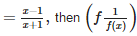equals

Solution:

f(x) = (x−1)/(x+1)
1/f(x) = (x+1)/(x-1)
f(1/f(x)) = [(x+1)/(x-1) - 1]/[(x+1)/(x-1) + 1]
⇒ (x+1-x+1)/(x+1+x-1)
= 2/2x
= 1/x

QUESTION: 2

### If A = {(x,y):x2+y2 = 25} and B = {(x,y) : x2+9y2+y2 = 144} then A∩B contains

Solution:

A is the set of all points on the circle x2 + y2 = 25 and B is the set of all points on the ellipse x2 + 9y2 = 144. These two intersect at four points P,Q,R and S.
Hence, A∩B contains four points.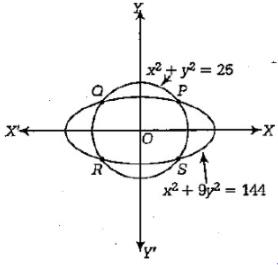QUESTION: 3

###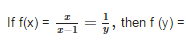Solution:
QUESTION: 4

The function f(x) = log (1+x/1-x) satisfies the equation

Solution:
QUESTION: 5

If f(x + y + z) = f(x) f(y) f(z) for all x , y z and if f(2) = 4, f’(0) = 5 and f(0) ≠ 0, then f’(2) is equal to

Solution: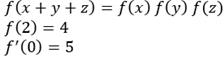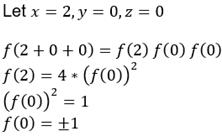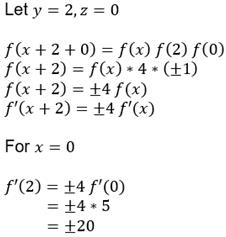QUESTION: 6

The domain of the function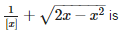Solution: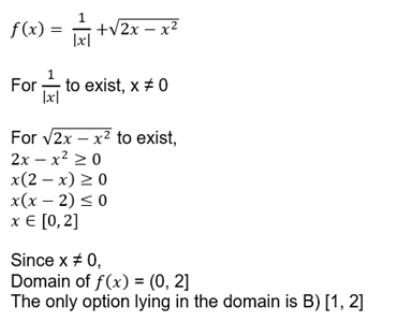QUESTION: 7

If A = {(x, y) : x2+y2 = 5} and B = {(x, y) : 2x = 5y}, then A∩B contains

Solution:

A = {(x, y) : x2+y2 = 5} B = {2x = 5y}
A is the set of all the points on a circle with centre (0, 0)
B is the set of all points on a line with slope 2/5 and passing through (0, 0)
So, the circle and the line intersect at two points.
So, A ∩ B contains two points

QUESTION: 8

If f : R → R is given by f(x) = |x| and A = {x∈R:x<0}, then f-1 (A) equals

Solution:
QUESTION: 9

If f : N × N →N is such that f (m, n) = m + n where N is the set of natural number, then which of the following is true ?

Solution:
QUESTION: 10

The function (sin x/3) is periodic with period

Solution:

sin(sinx/3)
As we know that {T : 2π}
T = (sinx/3)
2π = (sinx/3)
Period = 6π

QUESTION: 11

If A = [a, b], B = [c,d], C = [d, e] then {(a, c), (a, d), (a,e), (b,c), (b, d), (b, e)} is equal to

Solution:

Clearly, the set of first elements of ordered pairs in the given set is (a,b) and the set of second elements is {c, d, e}.
∴ {(a,c),(a,d),(a,e),(b,c),(b,d),(b,e)}
∴ {(a,c),(a,d),(a,e),(b,c),(b,d),(b,e)}
= {a,b}×{c,d,e}=A×(B∪C)

QUESTION: 12

The function f(x) = 10x from R to [0, ∞) is

Solution:
QUESTION: 13

For all x ∈ (0, 1)

Solution:
QUESTION: 14

The domain of the function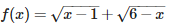Solution:

f(x) = (1-x)½ + (6-x)½
x-1≥0   6-x≥0
x≥1        x≤6
= [1,6]

QUESTION: 15

Let f (x) = x2 and g (x) = √x, then

Solution:
QUESTION: 16

Suppose that g (x) = 1+ √x and f(g(x)) = 3 + 2 √x + x, then f (x) is

Solution: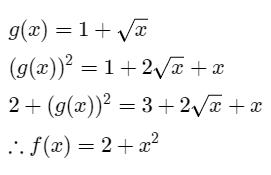QUESTION: 17

The number of elements in the Power set P(S) of the set S = [[Φ], 1, [2, 3]] is

Solution:

A = {1,2,3}
B : set of all subsets of A
List out all the subsets of A - {1},{2},{3},{1,2},{2,3},{1,3},{1,2,3},{empty set}
Number of elements in A (n) = 3 so 23 = 8
So, B = {{1},{2},{3},{1,2},{2,3},{1,3},{1,2,3},{empty set}} and the number of elements are 8.

QUESTION: 18

The minimum value of (x - α) (x – β) is

Solution: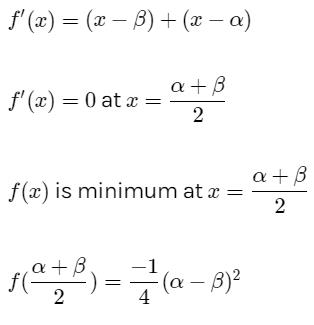QUESTION: 19

If f(x) = (25 - x4)1/4 for 0 < x < √5, then f(f(1/2)) =

Solution: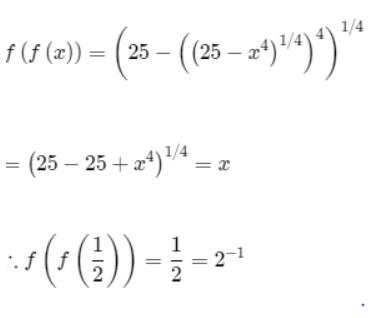QUESTION: 20

Two functions f:R→R g : R→Rare defined as follows :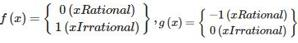then (gof)(e) + (fog)(π) =

Solution:
QUESTION: 21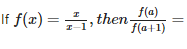Solution:
QUESTION: 22

Let function f : R → R be defined by f(x) = 2x + sin x for x ∈ R. Then f is

Solution:
QUESTION: 23

Coefficient of x3 in the expansion of tan x is

Solution:
QUESTION: 24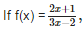then (fof) (2) is equal to

Solution:
QUESTION: 25

If f(x) = log (1+x/1-x), then f(x) is

Solution: